Courses

# RD Sharma Solutions Ex-13.2, Linear Equation In Two Variables, Class 9, Maths Class 9 Notes | EduRev

## Class 9 : RD Sharma Solutions Ex-13.2, Linear Equation In Two Variables, Class 9, Maths Class 9 Notes | EduRev

The document RD Sharma Solutions Ex-13.2, Linear Equation In Two Variables, Class 9, Maths Class 9 Notes | EduRev is a part of the Class 9 Course RD Sharma Solutions for Class 9 Mathematics.
All you need of Class 9 at this link: Class 9

Q 1 : Write two solutions for each of the following equations:

(i) 5x – 2y = 7

(ii) x = 6y

(iii) x +πy = 4

(iv) 2/3x – y = 4.

A 1 :

(i) We are given,

3x + 4y = 7

Substituting x = 1

In the given equation,

We get

3 x1 + 4y  = 7

4y = 7 – 3  4 = 4Y

Y = I

Thus x = 1 and y = 1 is the solution of 3x + 4y = 7

Substituting x = 2 in the given equation,

we get 3×2 + 4y = 7

4y = 7 – 6

y = 1/4

Thus x = 2 and  y = 1/4 is the solution of 3x + 4y = 7

(ii) We are given, x = 6y

Substituting x =0 in the given equation,

we get 0 = 6y

6 y = 0

y = 0

Thus x = 0,       ⇒ Solution (0,0)

Substituting x=6

6 = 6y

y =  6/6

y = 1          ⇒ Solution (6,1)

(iii)  We are given x+πy = 4

Substituting x = 0 in the given equation,

We get 0+πy = 4

πy = 4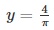⇒ Solution  =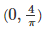Substituting y = 0 in the given equation, we get

x + 0 = 4

x = 4

⇒ Solution = (4, 0)

(iv) We are given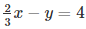Substituting x = 0 in the given equation, we get

0 – y = 4

y = – 4

Thus x = 0 and y = – 4  is a solution

Substituting x = 3 in the given equation, we get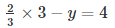2 – y = 4

y = 2 – 4

y = -2

Thus x = 3 and y = -2 is a solution

Q 2 : Write two solutions of the form x = 0,  y = a and x = b, y = 0 for each of the following  equations :

(i) 5x – 2y =10
(ii) -4x + 3y =12
(iii) 2x + 3y = 24

A 2 :

(i) We are given,

5x – 2y = 10

Substituting x = 0 in the given equation,

We get;

5×0 – 2y = 10

– 2y = 10

– y = 10/2

y = – 5

Thus x = 0 and y = -5 is the solution of 5x-2y = 10

Substituting y = 0 in the given equation, we get 5x —2 x 0 = 10

5x = 10

x = 10/2

x = 2

Thus x = 2 and y = 0 is a solution of 5x-2y = 10

(ii) We are given, – 4x + 3y = 12

Substituting x = 0 in the given equation,

we get;

-4×0 + 3y = 12

3y = 12

y = 4

Thus x = 0 and y = 4 is a solution of the -4x + 3y = 12

Substituting y = 0 in the given equation, we get;

-4 x + 3 x 0 = 12

– 4x = 12

x =-12/4

x = -3

Thus x = -3 and y =0 is a solution of -4x + 3y = 12

(iii) We are given, 2x + 3y = 24

Substituting x = 0 in the given equation, we get;

2 x 0 + 3y = 24

3y =24

y = 24/3

y = 8

Thus x = 0 and y = 8 is a solution of 2x+ 3y = 24

Substituting y = 0 in the given equation, we get;

2x +3 x 0 = 24

2x = 24

x = 24/2

x =12

Thus x =12 and y = 0 is a solution of 2x + 3y = 24

Q3: Check which of the following are solutions of the equation 2x — y = 6 and Which are not :

(i) (3 , 0)
(ii) (0 , 6)
(iii) (2 , – 2)
(iv)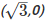(v)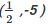A3:

We are given, 2x – y = 6

(i) In the equation 2 x – y = 6,

We have L.H.S = 2x — y and R.H.S = 6

Substituting x = 3 and y = 0 in 2x – y ,

We get L.H.S = 2 x 3 – 0 = 6

⇒ L.H.S = R.H.S

⇒ (3,0) is a solution of 2x — y = 6.

(ii) In the equation 2x — y = 6,

We have L.H.S = 2x— y and R.H.S = 6

Substituting x = 0 and y = 6 in 2x — y

We get L.H.S = 2 x 0 – 6 = – 6

⇒ L.H.S ≠ R.H.S

⇒ (0,6) is not a solution of 2x — y = 6.

(iii) In the equation 2x — y = 6,

We have L.H.S = 2x – y and R.H.S = 6

Substituting x = 2 and y = – 2 in 2x — y,

We get L.H.S = 2 x 2 – (-2) = 6

⇒ L.H.S = R.H.S

⇒ (2,-2) is a solution of 2x — y = 6.

(iv) In the equation 2x — y = 6,

We have L.H.S = 2x- y and R.H.S=6

Substituting x = √3 and y = 0 in 2x — y,

We get L.H.S = 2 x √3 – 0

⇒ L.H.S ≠ R.H.S

⇒(√3,0)is not a solution of 2x — y = 6.

(v) In the equation 2x — y = 6,

We have L.H.S = 2x – y and R.H.S = 6

Substituting x = 1/2 and y =  in 2x — y, we get

L.H.S = 2 x(1/2) – (-5)

⇒1 + 5 =  6

⇒  L.H.S = R.H.S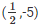is a solution of 2x — y = 6.

Q4:  If x = -1, y = 2 is a solution of the equation 3x + 4y = k, find the value of k.

A4 :

We are given, 3 x + 4 y = k

Given that, (-1,2) is the solution of equation 3x + 4y = k.

Substituting x = -1 and y = 2 in 3x + 4y = k,

We get; 3x – 1 + 4 x 2 = k

K = – 3 + 8

k = 5

Q 5 : Find the value of λ, if x = –λ and y = 5/2 is a solution of the equation x + 4y -7 = 0

A 5 :

We are given,

x + 4y – 7 = 0

(-λ,- 5)is a solution of equation 3x + 4y = k

Substituting x = – λ and y = 5/2 in x + 4y – 7 = 0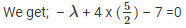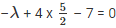λ = 10 – 7

λ = 3

Q 6 : If x = 2a + 1 and y = a -1 is a solution of the equation 2x – 3y + 5 = 0, find the value of a.

A 6:

We are given, 2x —3y +5 = 0

(2a + 1, a – 1) is the solution of equation 2x – 3y + 5 = 0.

Substituting x = 2a + 1 and y = a – 1 in 2x – 3y + 5 = 0,

We get 2 x 2a + (1- 3) x a – 1 + 5 = 0

⇒ 4a + 2 – 3a + 3 + 5 = 0

⇒ a + 10 = 0

⇒ a = – 10

Q 7 : If x = 1 and y = 6 is a solution of the equation 8x – ay + a2 = 0, find the values of a.

A 7 :

We are given,

8x – ay + a2 = 0

(1 , 6) is a solution of equation 8x — ay + a2 =0

Substituting x = 1 and y = 6 in 8x – ay + a2 =  0 ,we get

8 x 1 – a x 6 + a2 = 0

⇒ a2 – 6a + 8 = 0

a2 – 4a – 2a + 8 = 0

a(a – 4) – 2 (a – 4) = 0

(a – 2) (a – 4) = 0

a = 2, 4

Offer running on EduRev: Apply code STAYHOME200 to get INR 200 off on our premium plan EduRev Infinity!

91 docs

,

,

,

,

,

,

,

,

,

,

,

,

,

,

,

,

,

,

,

,

,

,

,

,

,

,

,

,

,

,

;